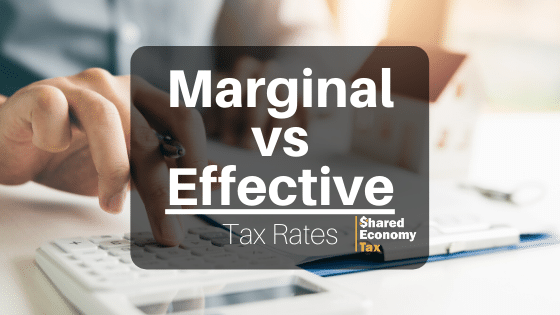Call us today: (888) 272 – 4915 (10am - 7pm CT)

## Accounting and Tax Tips Blog

Your tax rate determines how much you pay in taxes. There are currently 7 tax brackets, ranging from 10% to 35%, where you fit into the tax brackets will depend on your income. Sounds pretty straight forward, but there is actually a little more to it. The US has a progressive tax system, meaning the more you make the higher percentage you are taxed, however, just because your income fits into a certain tax bracket, does not mean you are taxed on your entire income. This is where marginal and effective tax rates come into play. You may be wondering what the difference is between marginal vs effective tax rate. Here we will explain.## What Is Effective Tax Rate

The effective tax rate represents the actual percentage of your annual income that you owe to the IRS. Also known as the average tax rate, effective tax rate measures how much federal income tax you pay on your earned income. The effective rate is usually lower than the marginal tax rate.

### How to Calculate Effective Tax Rate

While determining how much you owe in taxes can be a bit of a challenge, as a lot of things are taken into consideration, calculating your effective tax rate is pretty simple. To calculate your effective tax rate, simply divide your total amount of federal income tax paid, by your taxable income.

### Effective Tax Rate Formula

When calculating your effective tax rate you will use this formula:

• Income Tax ÷ Income Earned Before Taxes = Effective Tax Rate

For example, if you earned \$100,000 and paid \$25,000 in taxes, you would divide 25,000 by 100,000 and get an effective tax rate of .25%.

## What Is Marginal Tax Rate

The marginal tax rate measures the amount of tax applied on income that goes over the tax bracket limits. Tax brackets are progressive, so the IRS taxes income at different rates. This ensures that higher-income earners pay their fair share in taxes.

### Marginal Tax Rate Definition

Marginal tax rates apply to each additional dollar of income that surpasses your tax bracket’s income limit. Due to the marginal tax rate, you might have to pay different tax rates on your income.### How Do Marginal Tax Rates Work?

There are currently 7 tax brackets that range from 10% to 37%. Each bracket has an income limit, this limit varies depending on how you are filing; single, head of household, or jointly.

Your income may span several tax brackets, in which case you are taxed in each bracket until you reach the limit. This is the marginal tax rate.

For example, if you made \$32,000 and are filing as single, you would take the standard deduction of \$12,200. This would leave you with a taxable income of \$19,800. This spans the first two tax brackets, which means that your income would be taxed at 10% for the first \$9,700 and 12% for the remaining \$10,100. For higher-income earners, the process continues.

## What’s the Difference Between Marginal and Effective Tax Rate?

The difference between marginal vs effective tax rate is pretty simple. Effective tax rates are lower than marginal rates because they measure the actual tax rate you pay on your entire taxable income. Conversely, your marginal tax rate is varies based on your tax bracket.

### Do I Need to Know These Figures?

When it comes to your taxes, it is important to know where you stand. Knowing your effective tax rate tells you how much you are paying to the IRS. This can be useful for financial planning. Knowing your marginal tax rate can help with your tax planning strategy. It allows you to know what your tax liabilities will be with any additional income. This can help you plan and also strategize your tax deductions, which can save you money in the long run.